Download Presentation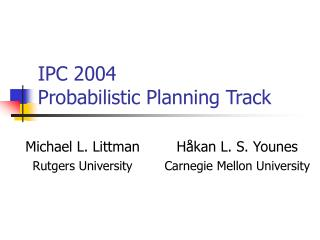IPC 2004 Probabilistic Planning Track

# IPC 2004 Probabilistic Planning Track - PowerPoint PPT Presentation

IPC 2004 Probabilistic Planning Track. Goal. Provide shared benchmarks and evaluation metrics for the MDP and probabilistic planning communities. Domain Model Restrictions. Discrete time Finite state space Full observability. Outline. Input language Competition problemsI am the owner, or an agent authorized to act on behalf of the owner, of the copyrighted work described.
Download Presentation## IPC 2004 Probabilistic Planning Track

An Image/Link below is provided (as is) to download presentation

Download Policy: Content on the Website is provided to you AS IS for your information and personal use and may not be sold / licensed / shared on other websites without getting consent from its author.While downloading, if for some reason you are not able to download a presentation, the publisher may have deleted the file from their server.

- - - - - - - - - - - - - - - - - - - - - - - - - - E N D - - - - - - - - - - - - - - - - - - - - - - - - - -
Presentation Transcript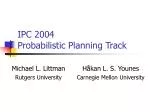### IPC 2004Probabilistic Planning Track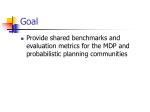Goal
• Provide shared benchmarks and evaluation metrics for the MDP and probabilistic planning communities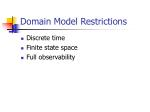Domain Model Restrictions
• Discrete time
• Finite state space
• Full observability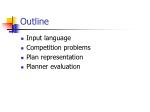Outline
• Input language
• Competition problems
• Plan representation
• Planner evaluation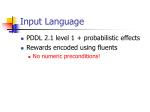Input Language
• PDDL 2.1 level 1 + probabilistic effects
• Rewards encoded using fluents
• No numeric preconditions!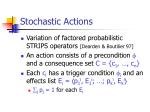Stochastic Actions
• Variation of factored probabilistic STRIPS operators [Dearden & Boutilier 97]
• An action consists of a precondition  and a consequence set C = {c1, …, cn}
• Each ci has a trigger condition i and an effects list Ei = p1i, E1i; …; pki, Eki
• j pj = 1 for each Ei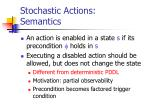Stochastic Actions:Semantics
• An action is enabled in a state s if its precondition holds in s
• Executing a disabled action should be allowed, but does not change the state
• Different from deterministic PDDL
• Motivation: partial observability
• Precondition becomes factored trigger condition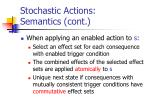Stochastic Actions:Semantics (cont.)
• When applying an enabled action to s:
• Select an effect set for each consequence with enabled trigger condition
• The combined effects of the selected effect sets are applied atomically to s
• Unique next state if consequences with mutually consistent trigger conditions have commutative effect sets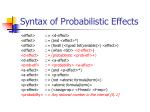Syntax of Probabilistic Effects

<effect> ::= <d-effect>

<effect> ::= (and <effect>*)

<effect> ::= (forall (<typed list(variable)>) <effect>)

<effect> ::= (when <GD> <d-effect>)

<d-effect> ::= (probabilistic <prob-eff>+)

<d-effect> ::= <a-effect>

<prob-eff> ::= <probability> <a-effect>

<a-effect> ::= (and <p-effect>*)

<a-effect> ::= <p-effect>

<p-effect> ::= (not <atomic formula(term)>)

<p-effect> ::= <atomic formula(term)>

<p-effect> ::= (<assign-op> <f-head> <f-exp>)

<probability> ::= Any rational number in the interval [0, 1]Correspondence to Components of Stochastic Actions
• Effects list:

(probabilistic p1i E1i … pki Eki)

• Consequence:

(when  (probabilistic p1i E1i … pki Eki))Stochastic Actions: Example

(:action stack

:parameters (?x ?y)

:precondition (and (holding ?x) (clear ?y))

:effect (and (not (holding ?x)) (clear ?x) (handempty)

(probabilistic 0.95 (and (not (clear ?y)) (on ?x ?y))

0.05 (ontable ?x))))Rewards and Goals
• Rewards encoded using fluents
• :effect (increase (reward) 100)
• (:metric maximize (reward))
• Meaning of goals
• (:goal ) means maximize probability of achieving Competition Problems
• Goal directed problems
• Fuzzy blocks world and logistics domains
• Reward directed problems
• Classical MDP type problemsDomain with Rewards

(define (domain tiger-domain)

(:requirements :negative-preconditions :conditional-effects :probabilistic-effects :rewards)

(:predicates (tiger-on-left) (hear-tiger-on-left))

(:action listen

:effect (and (when (tiger-on-left)

(probabilistic 0.85 (hear-tiger-on-left)

0.15 (not (hear-tiger-on-left))))

(when (not (tiger-on-left))

(probabilistic 0.85 (not (hear-tiger-on-left))

0.15 (hear-tiger-on-left)))))

(:action open-left-door

:effect (and (when (not (tiger-on-left)) (increase (reward) 100))

(when (tiger-on-left) (decrease (reward) 100))))

(:action open-right-door

:effect (and (when (tiger-on-left) (increase (reward) 100))

(when (not (tiger-on-left)) (decrease (reward) 100)))))MDP Planning Problem

(define (problem tiger-problem)

(:domain tiger-domain)

(:init (probabilistic 0.5 (tiger-on-left)))

(:metric maximize (reward))Plan Representation
• No explicit plan representation
• Up to each individual planner
• Planner communicates with evaluator
• Evaluator sends state updates to planner
• Planner sends actions choices to evaluator

Distinction between planner and executor blurredPlanner Evaluation
• Sampling-based planner evaluation
• Simulate execution for some time and accumulate reward
• Take average accumulated reward over multiple sample executions
• Three speed categories (preliminary)
• Real-time, intermediate, deliberative
• Variation in time allowed per sampleEvaluation Issues
• How many samples?
• How much time per sample?
• Require replanning for each sample, or allot initial computation time?Possible Subtracks
• Non-deterministic planning
• Treat probabilistic effects as disjunctive effects
• Learning
• Generalize from smaller problem instancesResources
• On the web:

http://www.cs.rutgers.edu/~mlittman/topics/ipc04-pt.html

• Mailing lists [mlittman@cs.rutgers.edu]:
• probplan-panel (discussion the design of the competition)
• probplan-announce (general announcements)